# UNIT IX: Atomic and Nuclear Physics - Online Test

Q1. Suppose an alpha particle accelerated by a potential of V volt is allowed to collide with a nucleus whose atomic number is Z, then the distance of closest approach of alpha particle to the nucleus is
Explaination / Solution: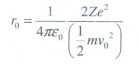When alpha particle is accelerated at the potential V;

K.E = 2eV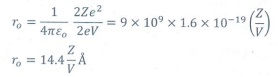Q2. In a hydrogen atom, the electron revolving in the fourth orbit, has angular momentum equal to
Explaination / Solution:

Angular momentum L = n h

For n = 4, L = 4h = 4h /2 π =2h/ π

Q3. Atomic number of H-like atom with ionization potential 122.4 V for n = 1 is
Explaination / Solution:

The ionization potential is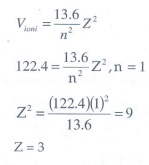Q4. The ratio between the first three orbits of hydrogen atom is
Explaination / Solution:

The radius of the nth orbit is rn  = n2r0

rn   n2

r1:r2:r3 = 1:4:9

Q5. The charge of cathode rays is
Explaination / Solution:

Cathode ray is a beam of electrons

Q6. In J.J. Thomson e/m experiment, a beam of electron is replaced by that of muons (particle with same charge as that of electrons but mass 208 times that of electrons). No deflection condition is achieved only if
Explaination / Solution: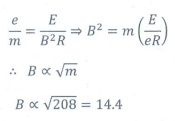Q7. The ratio of the wavelengths for the transition from n =2 to n = 1 in Li++, He+ and H is
Explaination / Solution: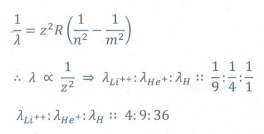Q8. The electric potential between a proton and an electron is given by V=V0ln ( r/r0 ) , where r0 is a constant. Assume that Bohr atom model is applicable to potential, then variation of radius of nth orbit rn with the principal quantum number n is
Explaination / Solution: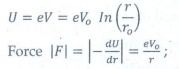It provide the centripetal force.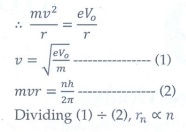Q9. If the nuclear radius of 27Al is 3.6 fermi, the approximate nuclear radius of 64Cu is
Explaination / Solution: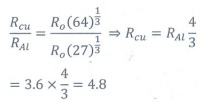Q10. The nucleus is approximately spherical in shape. Then the surface area of nucleus having mass number A varies as
Explaination / Solution:

Surface area = 4πR2

R=R0A1/3

Surface area = 4π(R0A1/3)2

area A2/3